# GMAT Math : DSQ: Calculating the area of a polygon

## Example Questions

### Example Question #31 : Polygons

What is the area of a parallelogram with four equal sides?

(1)  Each side is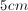(2)  One diagonal is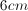Statement (1) ALONE is sufficient, but statement (2) alone is not sufficient.

EACH statement ALONE is sufficient.

BOTH statements TOGETHER are sufficient, but NEITHER statement ALONE is sufficient.

Statements (1) and (2) TOGETHER are NOT sufficient.

Statement (2) ALONE is sufficient, but statement (1) alone is not sufficient.

BOTH statements TOGETHER are sufficient, but NEITHER statement ALONE is sufficient.

Explanation: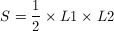(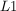and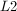are the diagonals of the rhombus). From statement (1) we cannot get to the lengths of diagonals. From statement (2) we only know the length of one diagonal, which is insufficient. However, putting the two statements together, we can use the Pythagorean Theorem to calculate the other diagonal, and then use the formula to calculate the area.

### Example Question #32 : Polygons

What is the area of a regular hexagon?

Statement 1: The perimeter of the hexagon is 48.

Statement 2: The radius of the hexagon is 8.

BOTH statements TOGETHER are sufficient to answer the question, but NEITHER statement ALONE is sufficient to answer the question.

Statement 1 ALONE is sufficient to answer the question, but Statement 2 ALONE is NOT sufficient to answer the question.

EITHER statement ALONE is sufficient to answer the question.

Statement 2 ALONE is sufficient to answer the question, but Statement 1 ALONE is NOT sufficient to answer the question.

BOTH statements TOGETHER are insufficient to answer the question.

EITHER statement ALONE is sufficient to answer the question.

Explanation:

A regular hexagon can be viewed as the composite of six equilateral triangles. The sidelength of each of the triangles is equal to both the sidelength of the hexagon (one-sixth of the perimeter) and the radius of the hexagon. From either statement, it is possible to find the area of one triangle by substituting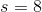in the area formula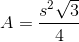and multiplying the result by 6.

### Example Question #33 : Polygons

What is the area of a trapezoid?

Statement 1: The length of its midsegment is 20.

Statement 2: The lengths of its bases are 18 and 22.

Statement 2 ALONE is sufficient to answer the question, but Statement 1 ALONE is NOT sufficient to answer the question.

Statement 1 ALONE is sufficient to answer the question, but Statement 2 ALONE is NOT sufficient to answer the question.

EITHER statement ALONE is sufficient to answer the question.

BOTH statements TOGETHER are sufficient to answer the question, but NEITHER statement ALONE is sufficient to answer the question.

BOTH statements TOGETHER are insufficient to answer the question.

BOTH statements TOGETHER are insufficient to answer the question.

Explanation:

The area of a trapezoid can be found using the following formula: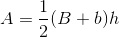Where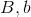are the lengths of the bases andis the height. From either statement,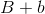can be determined, butis not given in either statement.

### Example Question #34 : Polygons

What is the area of a regular hexagon?

Statement 1: The area of the circle inscribed inside the hexagon is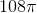.

Statement 2: The circumference of the circle that is circumscribed about the hexagon is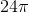.

Statement 2 ALONE is sufficient to answer the question, but Statement 1 ALONE is NOT sufficient to answer the question.

BOTH statements TOGETHER are insufficient to answer the question.

EITHER statement ALONE is sufficient to answer the question.

Statement 1 ALONE is sufficient to answer the question, but Statement 2 ALONE is NOT sufficient to answer the question.

BOTH statements TOGETHER are sufficient to answer the question, but NEITHER statement ALONE is sufficient to answer the question.

EITHER statement ALONE is sufficient to answer the question.

Explanation:

A regular hexagon can be viewed as a composite of six equilateral triangles, each of whose sidelength is the radius - the distance from its center to a vertex - of the hexagon. If the radius of the hexagonis known, then the area of the hexagon can be calculated to be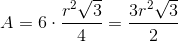.

From Statement 1 alone, the radius of the inscribed circle, or incircle, can be calculated from the area formula (by dividing the area byand extracting the square root). This length coincides with the height of each equilateral triangle. From there, the 30-60-90 Theorem can be used to find the sidelength of each triangle, and the area of the hexagon follows.

From Statement 2 alone, the radius of the circumscribed circle, or circumcircle, can be found by dividing its circumference by. This radius coincides with the radius of the hexagon, and the area can be calculated from there.

### Example Question #35 : Polygons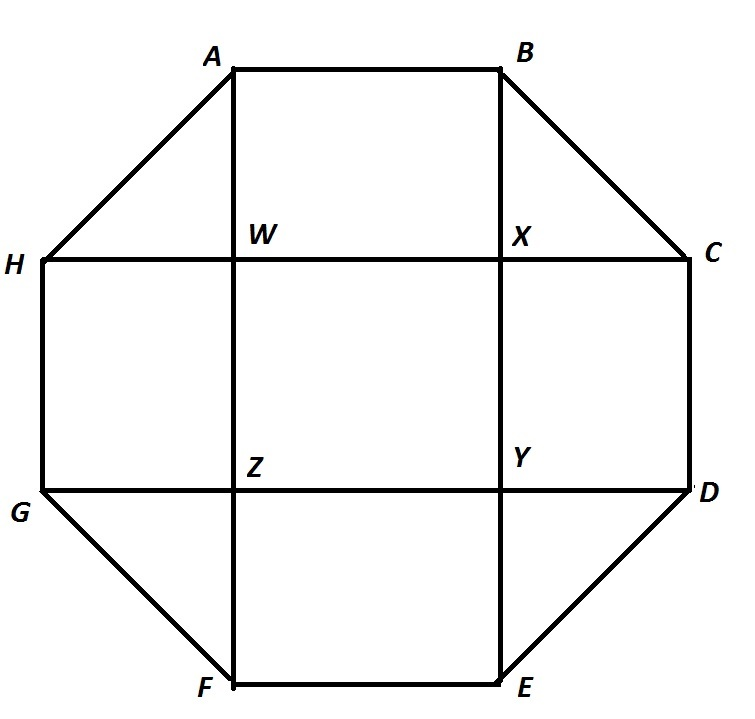Give the area of the above regular octagon.

Statement 1: The circle that circumscribes Quadrilateral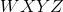has area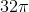.

Statement 2: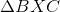has area 16.

BOTH statements TOGETHER are sufficient to answer the question, but NEITHER statement ALONE is sufficient to answer the question.

Statement 1 ALONE is sufficient to answer the question, but Statement 2 ALONE is NOT sufficient to answer the question.

EITHER statement ALONE is sufficient to answer the question.

Statement 2 ALONE is sufficient to answer the question, but Statement 1 ALONE is NOT sufficient to answer the question.

BOTH statements TOGETHER are insufficient to answer the question.

EITHER statement ALONE is sufficient to answer the question.

Explanation:

Quadrilateralis a square; each of its diagonals is a diameter of its circumscribed circle, or circumcircle. Therefore, if we know the area of its circumcircle from Statement 1 to be, we can calculate the radius from the area formula (divide by, extract the square root of the quotient). Twice this is the diameter, which is also the length of a diagonal of this square. Divide this byto get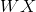. This is also equal to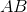, the length of one side; this is sufficient to get the area of the octagon.

From Statement 2 alone, since the area of isosceles triangleis known to be 8, the length of each leg can be found using the formula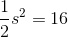Sinceis a 45-45-90 triangle, multiply this leg length byto get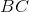, the length of one side; this is sufficient to get the area of the octagon.

Tired of practice problems?

Try live online GMAT prep today.